## Heat Conduction Equation

A diffusion equation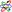of the form(1)

Physically, the equation commonly arises in situations whereis the thermal diffusivityand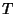the temperature.The 1-D heat conduction equation is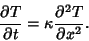(2)

This can be solved by Separation of Variables using(3)

Then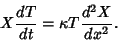(4)

Dividing both sides bygives(5)

where each side must be equal to a constant. Anticipating the exponential solution in, we have picked a negative separation constant so that the solution remains finite at all times and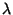has units of length. Thesolution is(6)

and thesolution is(7)

The general solution is then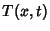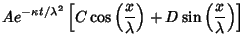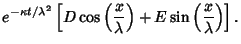(8)

If we are given the boundary conditions(9)

and(10)

then applying (9) to (8) gives(11)

and applying (10) to (8) gives(12)

so (8) becomes(13)

Since the general solution can have any,(14)

Now, if we are given an initial condition, we have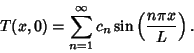(15)

Multiplying both sides byand integrating from 0 togives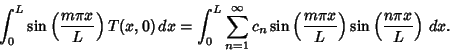(16)

Using the Orthogonality of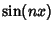and,(17)
so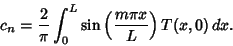(18)

If the boundary conditions are replaced by the requirement that the derivative of the temperature be zero at the edges, then (9) and (10) are replaced by(19)(20)

Following the same procedure as before, a similar answer is found, but with sine replaced by cosine: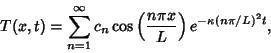(21)

where(22)